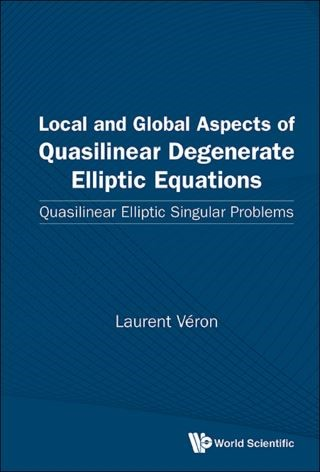## Local And Global Aspects Of Quasilinear Degenerate Elliptic Equations: Quasilinear Elliptic Singular Problems• Description
• Author
• Info
• Reviews

### Description

This book is devoted to the study of elliptic second-order degenerate quasilinear equations, the model of which is the p-Laplacian, with or without dominant lower order reaction term. Emphasis is put on three aspects:

• The existence of separable singular solutions enables the description of isolated singularities of general solutions. The construction of singular solutions is delicate and cannot be done without the understanding of the spherical p-harmonic eigenvalue problem.
• When the equations are considered on a Riemannian manifold, existence or non-existence of solutions depends on geometric assumptions such as the curvature. A priori estimates and Liouville type problems are analyzed.
• When the equations are considered with a forcing term in the class of measures, their study is strongly linked to the properties of a class of potentials appearing in harmonic analysis such as the Riesz, the Bessel or the Wolff potentials and to their associated capacities. Necessary and sufficient conditions for existence of solutions link the continuity of the measure with respect to some appropriate capacity.
Contents:
• Regularity
• Separable Solutions
• Quasilinear Equations with Absorption
• Quasilinear Equations with Measure Data
• Quasilinear Equations with Absorption and Measure Data
• Quasilinear Equations with Source

Readership: Graduate students and researchers interested in singularities of elliptic nonlinear equations.
p-Laplacian;Bessel;Riesz and Wolff Potentials;Singularities;Hamiltona€??Jacobi Equations;Measures and CapacitiesKey Features:
• The material presented is new and published in the last twenty years. If some rare topics are older, a new treatment is provided
• There will be a series of open problems at the end of each chapter